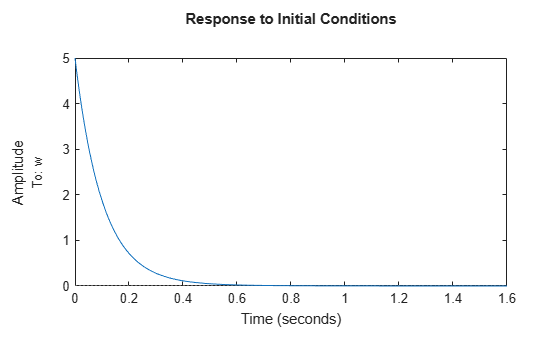Response from Initial Conditions

This example shows how to compute and plot the response of a state-space (ss) model to specified initial state values using initial.

sys_dc.InputName = 'Volts';
sys_dc.OutputName = 'w';
sys_dc.StateName = {'Current','w'};
sys_dc
sys_dc =

A =
Current        w
Current       -4    -0.03
w           0.75      -10

B =
Volts
Current      2
w            0

C =
Current        w
w        0        1

D =
Volts
w      0

Continuous-time state-space model.

This example uses the SISO, 2-state model sys_dc. This model represents a DC motor. The input is an applied voltage, and the output is the angular rate of the motor ω. The states of the model are the induced current $i$ (x1), and ω (x2). The model display in the command window shows the labeled input, output, and states.

Plot the undriven evolution of the motor's angular rate from an initial state in which the induced current is 1.0 amp and the initial rotation rate is 5.0 rad/s.

x0 = [1.0 5.0];
initial(sys_dc,x0)initial plots the time evolution from the specified initial condition on the screen. Unless you specify a time range to plot, initial automatically chooses a time range that illustrates the system dynamics.

Calculate the time evolution of the output and the states of sys_dc from $t$ = 0 (application of the step input) to $t$ = 1 s.

t = 0:0.01:1;
[y,t,x] = initial(sys_dc,x0,t);

The vector y contains the output at each time step in t. The array x contains the state values at each time step. Therefore, in this example x is a 2-by-101 array. Each row of x contains the values of the two states of sys_dc at the corresponding time step.